–ü–æ–∏—Å–∫ –¥–∞—Ç–∞—à–∏—Ç–∞ (1.687.043 –∫–æ–º–ø–æ–Ω–µ–Ω—Ç–æ–≤) –ì–¥–µ –∏—Å–∫–∞—Ç—å–≤ –Ω–∞–∏–º–µ–Ω–æ–≤–∞–Ω–∏–∏–≤ –æ–ø–∏—Å–∞–Ω–∏–∏### Datasheet: AO4603L (ATMEL Corporation)

Complementary Enhancement Mode Field Effect Transistor
–°–∫–∞—á–∞—Ç—å:PDFZIP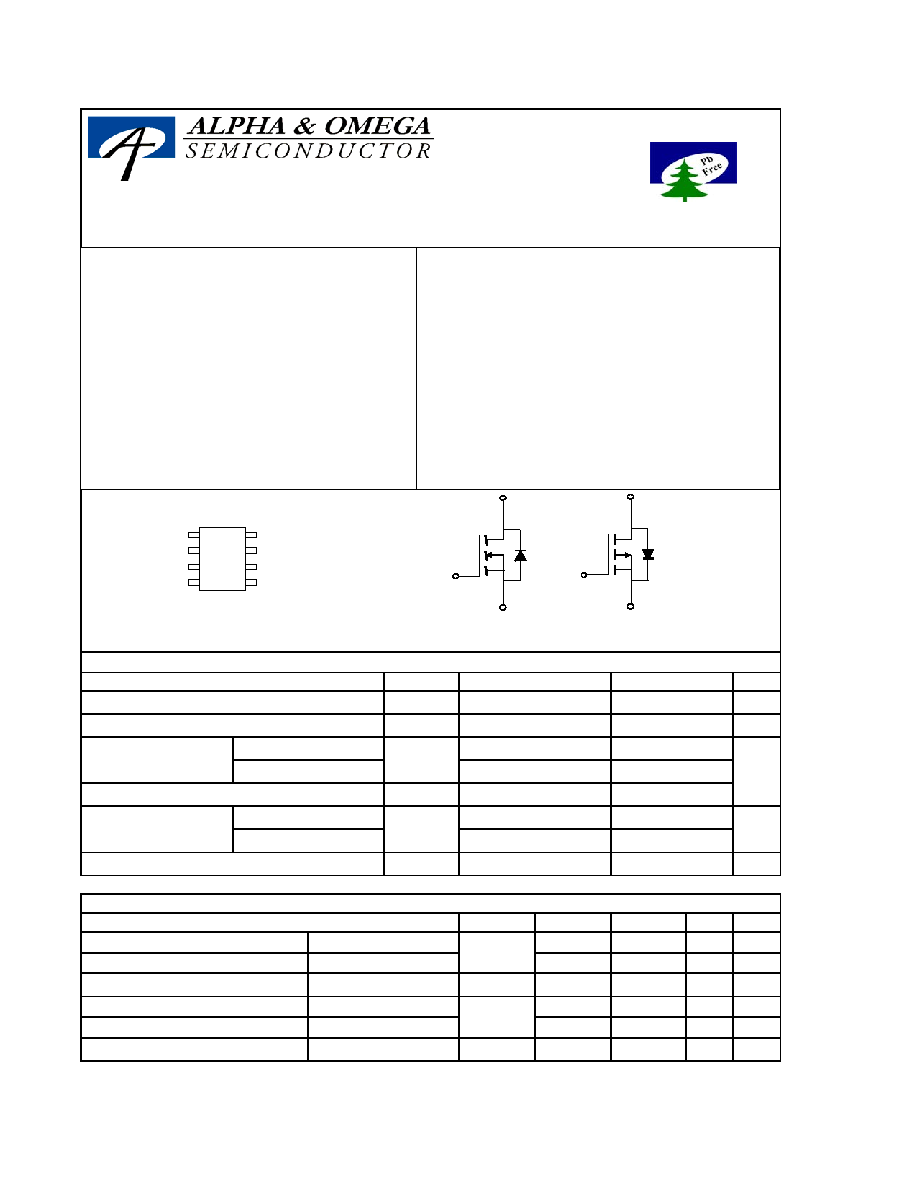Symbol
Max p-channel
Units
V
DS
V
V
GS
V
I
DM
T
J
, T
STG
∞C
Symbol
Device
Typ
Max Units
n-ch
52
62.5 ∞C/W
n-ch
78
110
∞C/W
R
JL
n-ch
48
50
∞C/W
p-ch
50
62.5 ∞C/W
p-ch
73
110
∞C/W
R
JL
p-ch
31
35
∞C/W
C
Maximum Junction-to-Ambient
A
t 10s
R
JA
Maximum Junction-to-Ambient
A
-40
T
A
=70∞C
Power Dissipation
T
A
=25∞C
P
D
Junction and Storage Temperature Range
A
Continuous Drain
Current
A
T
A
=25∞C
I
D
T
A
=70∞C
Pulsed Drain Current
B
W
4.7
4
30
2
1.44
-4.9
-5.8
2
1.44
Absolute Maximum Ratings T
A
=25∞C unless otherwise noted
Parameter
Max n-channel
30
-30
±20
Drain-Source Voltage
±12
Gate-Source Voltage
Thermal Characteristics: n-channel and p-channel
-55 to 150
-55 to 150
C
Parameter
Maximum Junction-to-Ambient
A
t 10s
R
JA
Maximum Junction-to-Ambient
A
AO4603
Complementary Enhancement Mode Field Effect Transistor
Features
n-channel p-channel
V
DS
(V) = 30V -30V
I
D
= 4.7A (V
GS
=10V) -5.8A (V
GS
=
-
10V)
R
DS(ON)
R
DS(ON)
< 55m
(V
GS
=10V) < 35m
(V
GS
=
-
10V)
< 70m
(V
GS
=4.5V) < 58m
(V
GS
=
-
4.5V)
< 110m
(V
GS
= 2.5V)
General Description
The AO4603 uses advanced trench technology
MOSFETs to provide excellent R
DS(ON)
and low
gate charge. The complementary MOSFETs
may be used to form a level shifted high side
switch, and for a host of other applications.
Standard product AO4603 is Pb-free (meets
ROHS & Sony 259 specifications). AO4603L is
a Green Product ordering option. AO4603 and
AO4603L are electrically identical.
G1
S1
G2
S2
D1
D1
D2
D2
1
2
3
4
8
7
6
5
SOIC-8
G2
D2
S2
G1
D1
S1
n-channel
p-channel
Alpha & Omega Semiconductor, Ltd.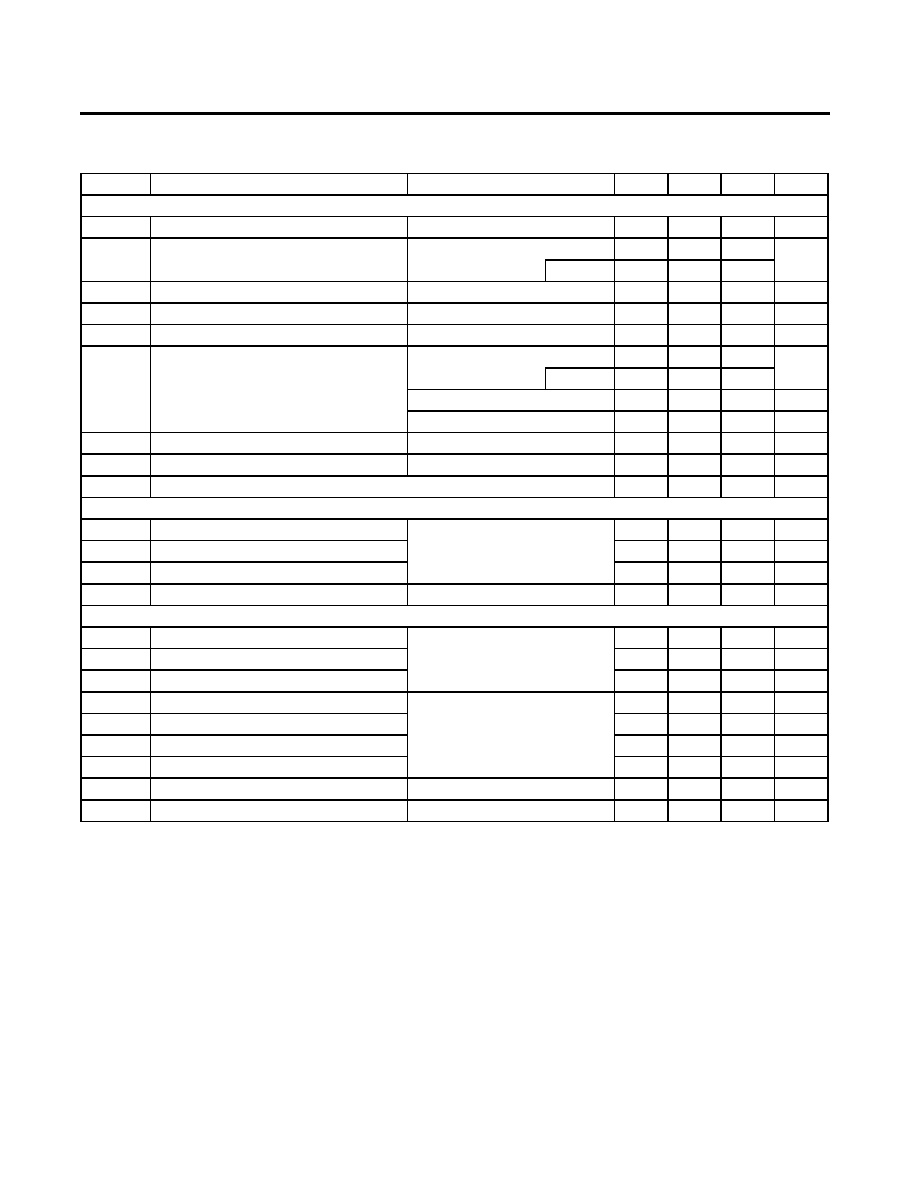AO4603
Symbol
Min
Typ
Max
Units
BV
DSS
30
V
1
T
J
=55∞C
5
I
GSS
100
nA
V
GS(th)
0.6
1
1.4
V
I
D(ON)
10
A
45
55
T
J
=125∞C
55
70
m
83
110
m
g
FS
8
S
V
SD
0.8
1
V
I
S
2.5
A
C
iss
390
pF
C
oss
54.5
pF
C
41
pF
R
g
3
Q
g
0.6
nC
Q
gs
1.38
nC
Q
gd
4.34
nC
t
D(on)
3.3
ns
t
r
1
ns
t
D(off)
21.7
ns
t
f
2.1
ns
t
rr
12
ns
Q
rr
6.3
nC
THIS PRODUCT HAS BEEN DESIGNED AND QUALIFIED FOR THE CONSUMER MARKET. APPLICATIONS OR USES AS CRITICAL
COMPONENTS IN LIFE SUPPORT DEVICES OR SYSTEMS ARE NOT AUTHORIZED. AOS DOES NOT ASSUME ANY LIABILITY ARISING
OUT OF SUCH APPLICATIONS OR USES OF ITS PRODUCTS. AOS RESERVES THE RIGHT TO IMPROVE PRODUCT DESIGN,
FUNCTIONS AND RELIABILITY WITHOUT NOTICE.
Body Diode Reverse Recovery Charge
V
GS
=0V, V
DS
=0V, f=1MHz
SWITCHING PARAMETERS
Total Gate Charge
V
GS
=4.5V, V
DS
=15V, I
D
=4A
I
F
=4A, dI/dt=100A/
µs
Gate resistance
Turn-Off DelayTime
Turn-Off Fall Time
Body Diode Reverse Recovery Time
Diode Forward Voltage
I
S
=1A,V
GS
=0V
Output Capacitance
Reverse Transfer Capacitance
Maximum Body-Diode Continuous Current
DYNAMIC PARAMETERS
Input Capacitance
V
GS
=0V, V
DS
=15V, f=1MHz
n-channel MOSFET Electrical Characteristics (T
J
=25∞C unless otherwise noted)
Parameter
Conditions
STATIC PARAMETERS
Drain-Source Breakdown Voltage
I
D
=250
µA, V
GS
=0V
I
DSS
Zero Gate Voltage Drain Current
V
DS
=24V, V
GS
=0V
µA
Gate-Body leakage current
V
DS
=0V, V
GS
=±12V
m
V
GS
=4.5V, I
D
=3A
Gate Threshold Voltage
V
DS
=V
GS
I
D
=250
µA
On state drain current
V
GS
=4.5V, V
DS
=5V
V
GS
=2.5V, I
D
=2A
V
DS
=5V, I
D
=4A
R
DS(ON)
Static Drain-Source On-Resistance
V
GS
=10V, I
D
=4A
Forward Transconductance
V
GS
=10V, V
DS
=15V, R
L
=3.75
,
R
GEN
=6
I
F
=4A, dI/dt=100A/
µs
Gate Source Charge
Gate Drain Charge
Turn-On DelayTime
Turn-On Rise Time
A: The value of R
JA
is measured with the device mounted on 1in
2
FR-4 board with 2oz. Copper, in a still air environment with T
A
=25∞C. The
value in any given application depends on the user's specific board design. The current rating is based on the t
10s thermal resistance rating.
B: Repetitive rating, pulse width limited by junction temperature.
C. The R
JA
is the sum of the thermal impedence from junction to lead R
JL
D. The static characteristics in Figures 1 to 6 are obtained using 80
µs pulses, duty cycle 0.5% max.
E. These tests are performed with the device mounted on 1 in
2
FR-4 board with 2oz. Copper, in a still air environment with T
A
=25∞C. The SOA
curve provides a single pulse rating.
Rev3: August 2005
Alpha & Omega Semiconductor, Ltd.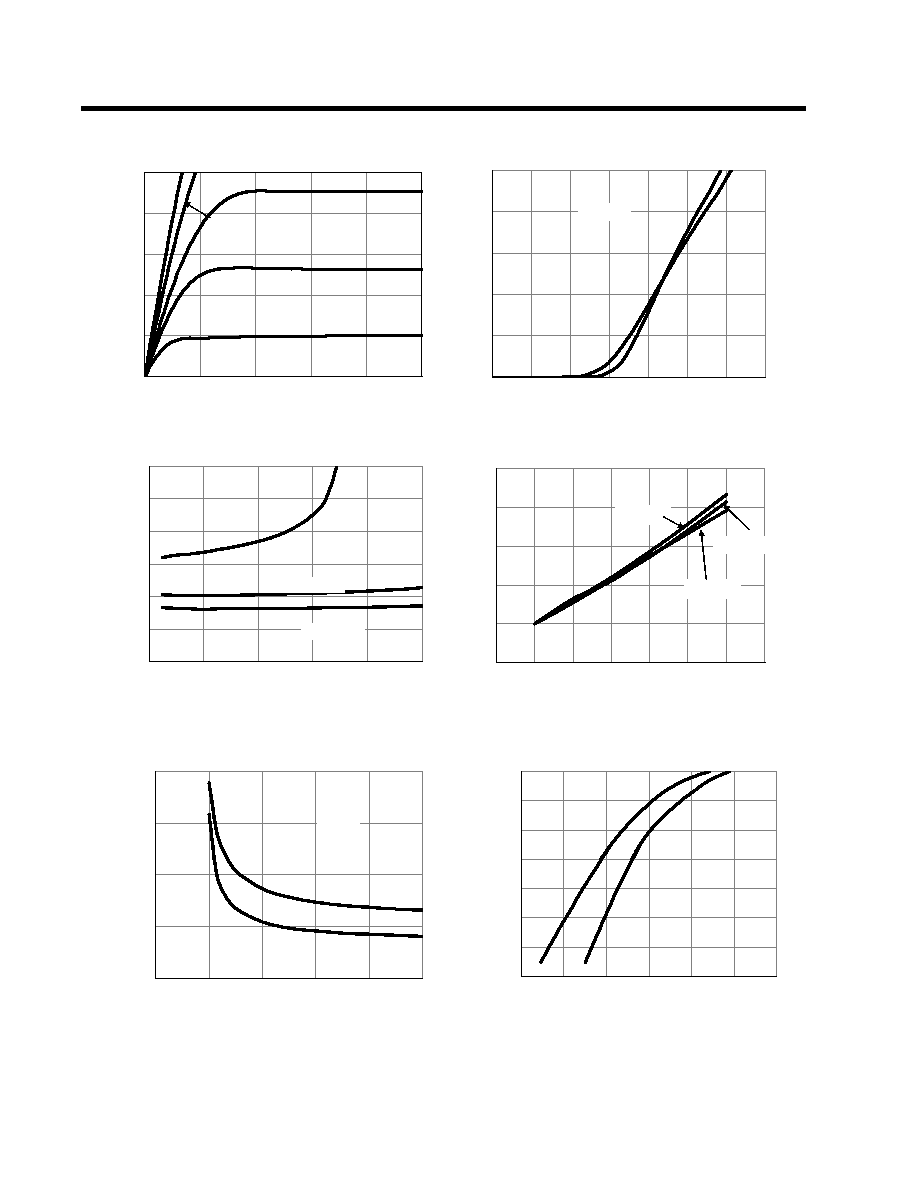AO4603
TYPICAL ELECTRICAL AND THERMAL CHARACTERISTICS
N-Channel
0
3
6
9
12
15
0
1
2
3
4
5
V
DS
(Volts)
Fig 1: On-Region Characteristics
I
D
(A
)
V
GS
=2V
2.5V
3V
4.5V
10V
0
2
4
6
8
10
0
0.5
1
1.5
2
2.5
3
3.5
V
GS
(Volts)
Figure 2: Transfer Characteristics
I
D
(A
)
0
25
50
75
100
125
150
0
2
4
6
8
10
I
D
(A)
Figure 3: On-Resistance vs. Drain Current and
Gate Voltage
R
DS
(
O
N)
(m
)
1.0E-06
1.0E-05
1.0E-04
1.0E-03
1.0E-02
1.0E-01
1.0E+00
1.0E+01
0.0
0.2
0.4
0.6
0.8
1.0
1.2
V
SD
(Volts)
Figure 6: Body-Diode Characteristics
I
S
(A
)
25∞C
125∞C
0.8
1
1.2
1.4
1.6
1.8
0
25
50
75
100
125
150
175
Temperature (∞C)
Figure 4: On-Resistance vs. Junction
Temperature
N
o
r
m
aliz
ed
On
-R
esistan
ce
V
GS
=2.5V
V
GS
=10V
V
GS
=4.5V
0
50
100
150
200
0
2
4
6
8
10
V
GS
(Volts)
Figure 5: On-Resistance vs. Gate-Source Voltage
R
DS
(
O
N)
(m
)
25∞C
125∞C
V
DS
=5V
V
GS
=2.5V
V
GS
=4.5V
V
GS
=10V
I
D
=2A
25∞C
125∞C
Alpha & Omega Semiconductor, Ltd.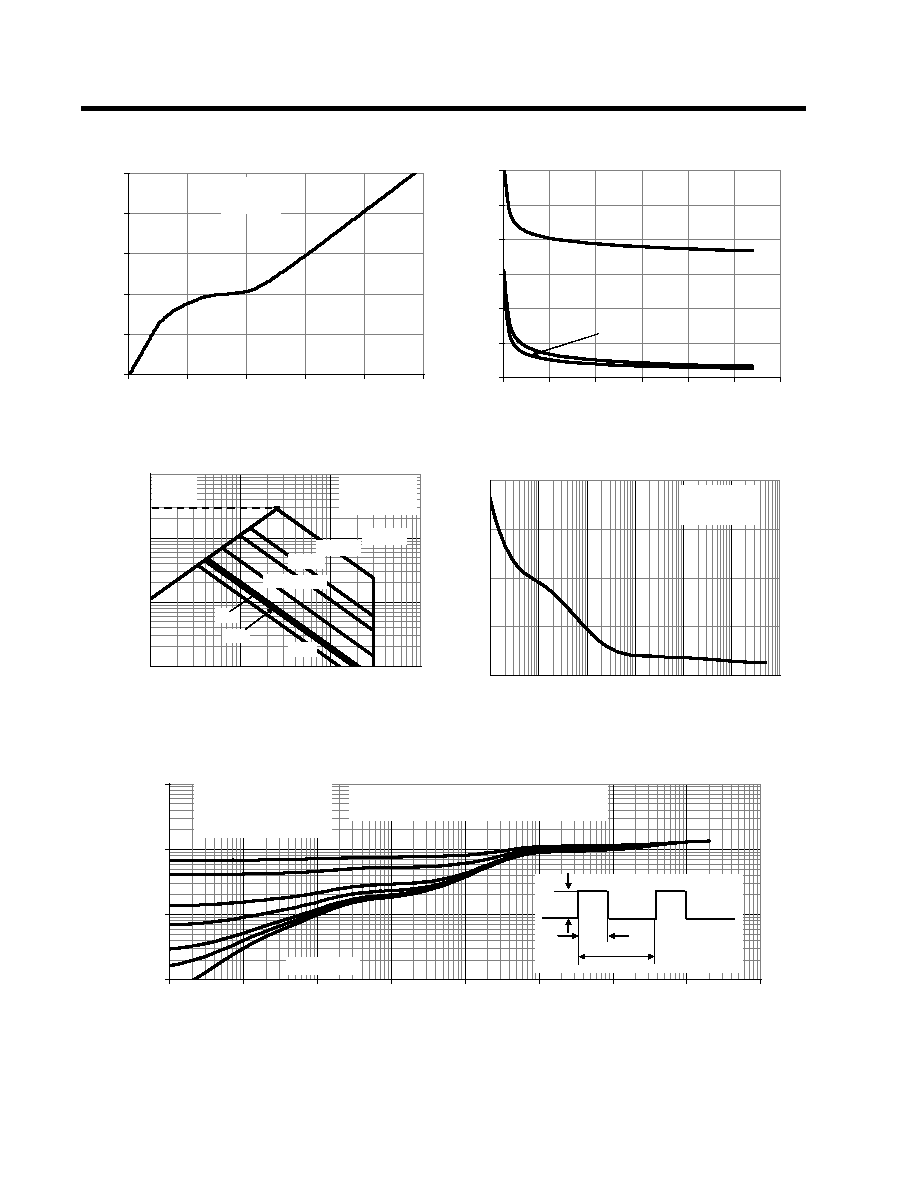AO4603
TYPICAL ELECTRICAL AND THERMAL CHARACTERISTIC
S N-Channel
0
1
2
3
4
5
0
1
2
3
4
5
Q
g
(nC)
Figure 7: Gate-Charge Characteristics
V
GS
(V
ol
ts)
0
100
200
300
400
500
600
0
5
10
15
20
25
30
V
DS
(Volts)
Figure 8: Capacitance Characteristics
C
a
pa
c
i
ta
nc
e
(pF)
C
iss
0
5
10
15
20
0.001
0.01
0.1
1
10
100
1000
Pulse Width (s)
Figure 10: Single Pulse Power Rating Junction-to-
Ambient (Note E)
Po
w
e
r (
W
)
0.01
0.1
1
10
0.00001
0.0001
0.001
0.01
0.1
1
10
100
1000
Pulse Width (s)
Figure 11: Normalized Maximum Transient Thermal Impedance
Z
JA
N
o
r
m
a
liz
ed
T
r
an
sien
t
T
h
er
m
al R
esistan
ce
C
oss
C
0.1
1.0
10.0
100.0
0.1
1
10
100
V
DS
(Volts)
I
D
(A
m
p
s)
Figure 9: Maximum Forward Biased Safe
Operating Area (Note E)
100
µs
10ms
1ms
0.1s
1s
10s
DC
R
DS(ON)
limited
T
J(Max)
=150∞C
T
A
=25∞C
V
DS
=15V
I
D
=4A
Single Pulse
D=T
on
/T
T
J,PK
=T
A
+P
DM
.Z
JA
.R
JA
R
JA
=90∞C/W
T
on
T
P
D
In descending order
D=0.5, 0.3, 0.1, 0.05, 0.02, 0.01, single pulse
T
J(Max)
=150∞C
T
A
=25∞C
10
µs
Alpha & Omega Semiconductor, Ltd.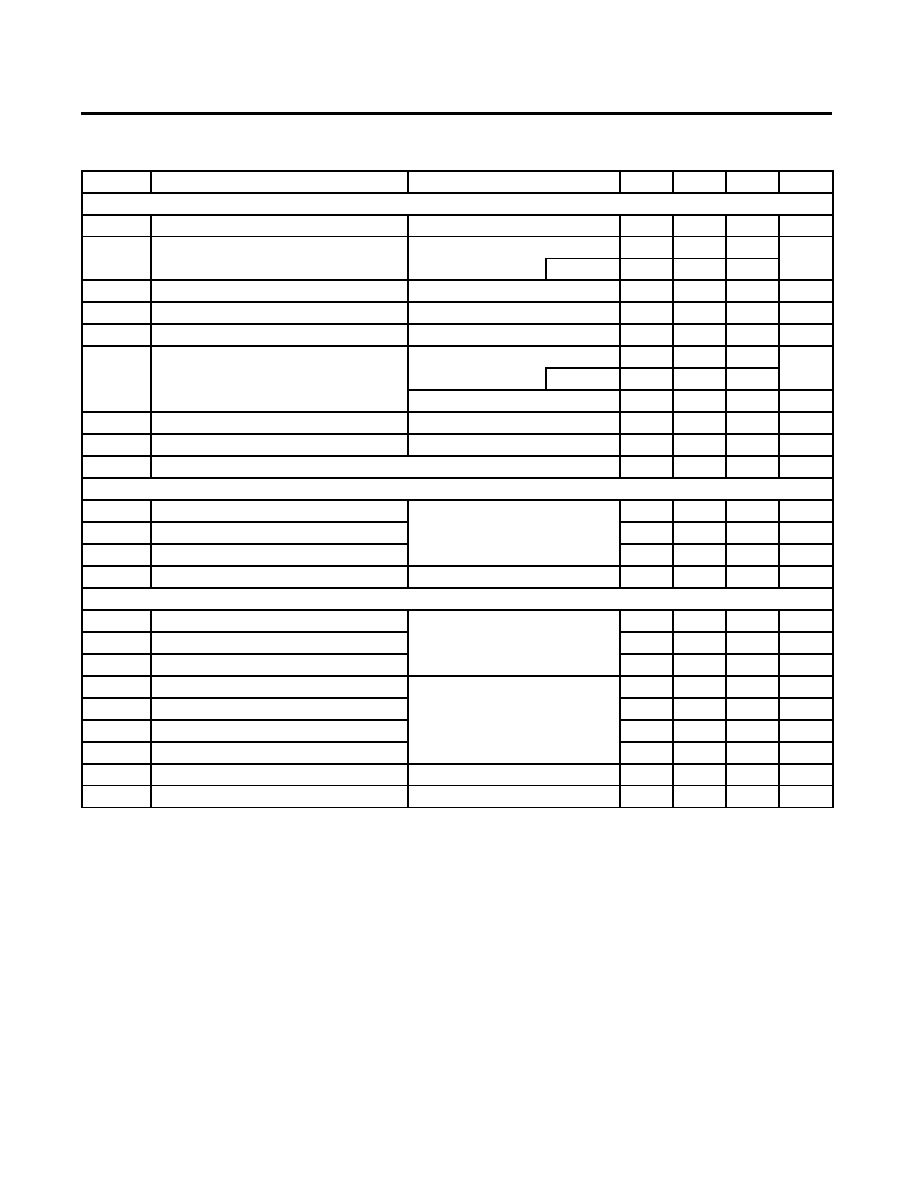AO4603
Symbol
Min
Typ
Max
Units
BV
DSS
-30
V
-1
T
J
=55∞C
-5
I
GSS
±100
nA
V
GS(th)
-1.2
-1.8
-2.2
V
I
D(ON)
40
A
29
38
T
J
=125∞C
40
39
63
m
g
FS
S
V
SD
-0.75
-1
V
I
S
-4.2
A
C
iss
920
pF
C
oss
190
pF
C
122
pF
R
g
3.6
Q
g
2.4
nC
Q
gs
4.5
nC
Q
gd
9.3
nC
t
D(on)
7.6
ns
t
r
5.2
ns
t
D(off)
21.6
ns
t
f
8
ns
t
rr
20
ns
Q
rr
8.8
nC
THIS PRODUCT HAS BEEN DESIGNED AND QUALIFIED FOR THE CONSUMER MARKET. APPLICATIONS OR USES AS CRITICAL
COMPONENTS IN LIFE SUPPORT DEVICES OR SYSTEMS ARE NOT AUTHORIZED. AOS DOES NOT ASSUME ANY LIABILITY ARISING
OUT OF SUCH APPLICATIONS OR USES OF ITS PRODUCTS. AOS RESERVES THE RIGHT TO IMPROVE PRODUCT DESIGN,
FUNCTIONS AND RELIABILITY WITHOUT NOTICE.
Body Diode Reverse Recovery Charge I
F
=-7.5A, dI/dt=100A/
µs
Turn-On Rise Time
Turn-Off DelayTime
Turn-Off Fall Time
Body Diode Reverse Recovery Time
V
GS
=-10V, V
DS
=-15V, R
L
=2
,
R
GEN
=3
I
F
=-7.5A, dI/dt=100A/
µs
Gate Source Charge
Gate Drain Charge
Turn-On DelayTime
SWITCHING PARAMETERS
Total Gate Charge
V
GS
=-10V, V
DS
=-15V, I
D
=-7.5A
Output Capacitance
Reverse Transfer Capacitance
Gate resistance
DYNAMIC PARAMETERS
Input Capacitance
V
GS
=0V, V
DS
=-15V, f=1MHz
V
GS
=0V, V
DS
=0V, f=1MHz
Diode Forward Voltage
I
S
=-1A,V
GS
=0V
Maximum Body-Diode Continuous Current
V
GS
=-10V, I
D
=-5A
m
V
GS
=-4.5V, I
D
=-5A
V
DS
=-5V, I
D
=-10A
R
DS(ON)
Static Drain-Source On-Resistance
Forward Transconductance
Gate Threshold Voltage
V
DS
=V
GS
I
D
=-250
µA
On state drain current
V
GS
=-10V, V
DS
=-5V
µA
Gate-Body leakage current
V
DS
=0V, V
GS
=±20V
Drain-Source Breakdown Voltage
I
D
=-250
µA, V
GS
=0V
I
DSS
Zero Gate Voltage Drain Current
V
DS
=-24V, V
GS
=0V
p-channel MOSFET Electrical Characteristics (T
J
=25∞C unless otherwise noted)
Parameter
Conditions
STATIC PARAMETERS
A: The value of R
JA
is measured with the device mounted on 1in
2
FR-4 board with 2oz. Copper, in a still air environment with T
A
=25∞C. The
value in analication depends on the user's specific board design. The current rating is based on the t
10s thermal resistance
rating.
B: Repetitive rating, pulse width limited by junction temperature.
C. The R
JA
is the sum of the thermal impedence from junction to lead R
JL
D. The static characteristics in Figures 1 to 6,12,14 are obtained using 80
µs pulses, duty cycle 0.5% max.
E. These tests are performed with the device mounted on 1 in
2
FR-4 board with 2oz. Copper, in a still air environment with T
A
=25∞C. The
SOA curve provides a single pulse rating.
A: The value of R
JA
is measured with the device mounted on 1in
2
FR-4 board with 2oz. Copper, in a still air environment with T
A
=25∞C. The
value in any a given application depends on the user's specific board design. The current rating is based on the t
10s thermal resistance
rating.
B: Repetitive rating, pulse width limited by junction temperature.
C. The R
JA
is the sum of the thermal impedence from junction to lead R
JL